# Pisot number

A real algebraic integer, all of whose other Galois conjugates have absolute value strictly less than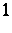(cf. also Galois theory). That is,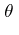satisfies a polynomial equation of the form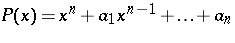, where theare integers,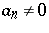and the roots of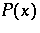other thanall lie in the open unit circle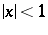. The set of these numbers is traditionally denoted by. Every positive integeris a Pisot number, but a more interesting example is the golden ratio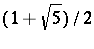. Every real number field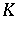contains infinitely many Pisot numbers of degree equal to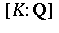, and, in fact, every real number fieldcan be generated by Pisot numbers, even by Pisot units (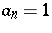).
The Pisot numbers have the interesting property that if, then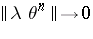as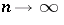, where here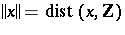denotes the distance from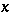to the nearest integer. It is an open question whether this property characterizes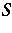among the real numbers(Pisot's conjecture). An important result of Ch. Pisot in this direction is that ifandare real numbers for which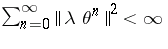, then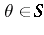and[a1].
The unusual behaviour of the powers of Pisot numbers leads to applications in harmonic analysis, [a3], [a5], dynamical systems theory (cf. also Dynamical system) [a6] and the theory of quasi-crystals [a4]. For example, if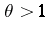, then the set of powers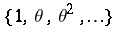is harmonious if and only ifis a Pisot number or a Salem number [a3]. The Bragg spectrum of the diffraction pattern of a self-similar tiling (cf., e.g., Voronoi lattice types) is non-trivial if and only if the scaling factor of the tiling is a Pisot number [a4].
A surprising fact is thatis a closed and hence nowhere-dense subset of the real line [a5]. The derived setsare all non-empty andas. (Heredenotes the set of limit points of,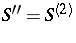the set of limit points of, etc., cf. also Limit point of a set). The order type ofis described in [a2]. The smallest elements of,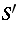and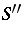are explicitly known [a1].
There is an intimate relationship between the setof Pisot numbers and the set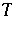of Salem numbers. It is known that, cf. Salem number. It seems reasonable to conjecture that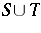is closed and that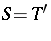, but it is not yet known whether or notis dense in.